# Triangles - Same Base, Same Parallels

Go back to  'Triangles-Quadrilaterals'

Consider two triangles on the same base and between the same parallels, as shown below: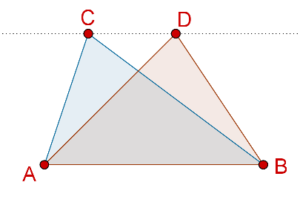The area of each triangle is half of the area of any parallelogram on the same base and between the same parallels. Thus, the area of the two triangles is the same. Let us formalize this as a theorem:

Theorem: Two triangles on the same base and between the same parallels are equal in area.

Also, we have already seen how to calculate the area of any triangle. Consider a triangle. Take side BC to be the base of this triangle. Now, measure the height of this triangle, which will be the distance between BC and the parallel to BC through A: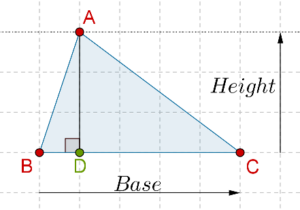In other words, the height of this triangle will be the length of the perpendicular AD (from A to BC). Thus, the area of this triangle can be written as:

A = ½ × BC × AD

Note that there is nothing special about BC – we can take any other side as the base as well. Let us take AC as the base. In that case, the height of the triangle will be the length of the perpendicular from B to AC: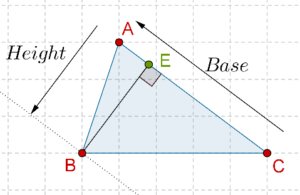The area of the triangle can now be written as:

A = ½ × AC × BE

Example 1: O is any point in the interior of a parallelogram ABCD. Show that:

area($$\Delta AOB$$) + area($$\Delta COD$$)

= area($$\Delta AOD$$) + area($$\Delta BOC$$)

= ½ area(ABCD)

Solution: Consider the following figure, which shows O to be any point inside the parallelogram ABCD. We have a draw a line through O which is parallel to AB: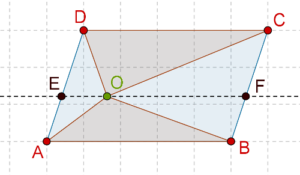We note that ABFE and CDEF are themselves parallelograms (why?). Now, the area of $$\Delta AOB$$ will be half of the area of parallelogram ABFE, because they are on the same base AB and between the same parallels AB and EF:

area($$\Delta AOB$$) = ½ area(ABFE)

Similarly, the area of $$\Delta COD$$ will be half of the area of parallelogram CDEF, because they are on the same base CD and between the same parallels CD and FE:

area($$\Delta COD$$) = ½ area(CDEF)

Thus,

area($$\Delta AOB$$) + area($$\Delta AOB$$)

= ½ {area(ABFE) + area(CDEF)}

= ½ area(ABCD)

We have shown that the areas of $$\Delta AOB$$ and $$\Delta COD$$ add up to half of the area of the parallelogram ABCD. The remaining area is the sum of the areas of $$\Delta AOD$$ and $$\Delta BOC$$. It is now obvious that this sum will also be equal to ½ area(ABCD).

Example 2: In what ratio (of areas) does any median divide a triangle?

Solution: Consider the following figure, in which AD is the median through A, and we have drawn a line through A parallel to BC: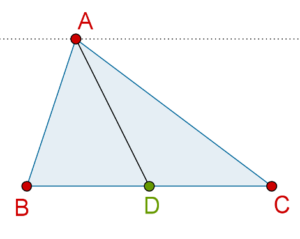Clearly, $$\Delta ABD$$ and $$\Delta ADC$$ are on equal bases (BD = DC), and between the same parallels. This means that their areas must be equal. Thus, any median will of a triangle will divide into two triangles of equal areas.

Example 3: Suppose that E is the midpoint of the median AD in $$\Delta ABC$$. What is the ratio of the areas of $$\Delta BED$$ and $$\Delta ABC$$?

Solution: Consider the following figure: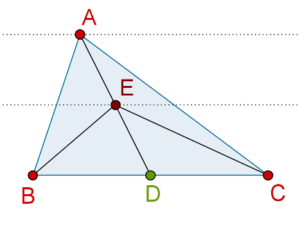We note that area($$\Delta BED$$) = area($$\Delta CED$$), because these triangles have equal bases (BD = DC) and they are between the same parallels.

Note also that E is the midpoint of AD, so that AE = ED. This means that area($$\Delta ABE$$) = area($$\Delta DBE$$), since these triangles are on equal bases (AE = ED) and between the same parallels (they have the same third vertex, point B). Similarly, area($$\Delta ACE$$) = area($$\Delta DCE$$). Thus, we have:

area($$\Delta BED$$) = area($$\Delta CED$$) = area($$\Delta AEB$$) = area($$\Delta AEC$$)

In other words, the areas of the four smaller triangles are equal. Also, the areas of these four triangles must add up to the total area of area($$\Delta ABC$$). Therefore,

area($$\Delta BED$$) = area($$\Delta CED$$) = area($$\Delta AEB$$) = area($$\Delta AEC$$) = area($$\Delta ABC$$)/4

The required ratio is 1:4.

Example 4: Consider the following figure, in which ABCD is a parallelogram. BC has been produced to Q, such that AD = CQ. AQ intersects CD at P: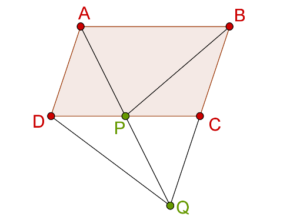Show that area($$\Delta BPC$$) = area($$\Delta DPQ$$).

Solution: We join AC, as shown below: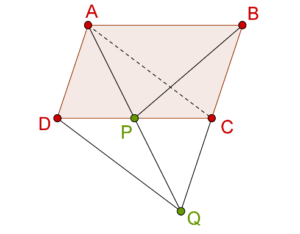Clearly, ACQD is a parallelogram (prove it!), so that P is the midpoint of AQ and CD. Thus,

area($$\Delta DPQ$$) = area($$\Delta APC$$)

We also note that $$\Delta APC$$ and $$\Delta BPC$$ are on the same base PC and between the same parallels AB and CD, so that:

area($$\Delta APC$$) = area($$\Delta BPC$$)

From the two relations above, we have:

area($$\Delta DPQ$$) = area($$\Delta BPC$$)